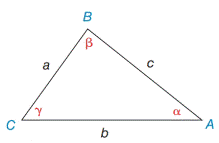Chapter 11.4, Problem 7EElementary Geometry For College St...

7th Edition
Alexander + 2 others
ISBN: 9781337614085

Solutions

Chapter
SectionElementary Geometry For College St...

7th Edition
Alexander + 2 others
ISBN: 9781337614085
Textbook Problem

In Exercises 7 and 8, state the form of the Law of Sines or the Law of Cosines that you would use to solve the problem. See the figure for Exercises 1 to 8.a) Find α if you know the values of a, b, and β .b) Find α if you know the values of a, b, and c.Exercises 1-8

To determine

a.

To Find:

The angle of α with the sides a, b and the angle β.

Explanation

Formula:

The Law of Sines,

sinαa=sinβb=sinγc

Where α, β, and γ is the acute angle of the triangle, and a, b, and c is the sides of the triangle.

Calculation:

Given,

The dimension of the triangle ABC a, b and angle β.

Here we have two sides and one angle so we use the Law of Sines to find α.

By the Law of Sines,

sinαa=sinβb=sinγc

Take,

sinαa=sinβb

Multiply a on both sides,

a(sin

To determine

b.

To find:

The angle of α with the sides a, b, and c.

Still sussing out bartleby?

Check out a sample textbook solution.

See a sample solution

The Solution to Your Study Problems

Bartleby provides explanations to thousands of textbook problems written by our experts, many with advanced degrees!

Get Started

In Problems 15-28, find the general solution to the given differential equation.

Mathematical Applications for the Management, Life, and Social Sciences

In Exercises 7-28, perform the indicated operations and simplify each expression. 16. x1x+2x+3x21

Applied Calculus for the Managerial, Life, and Social Sciences: A Brief Approach

Evaluate the integral. 2. 12x(x+1)2dx

Single Variable Calculus: Early Transcendentals

0 1 does not exist

Study Guide for Stewart's Single Variable Calculus: Early Transcendentals, 8th